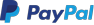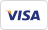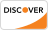Google's TensorFlow is an open-source and most popular deep learning library for research and production. This ebook covers basics to advance topics like linear regression, classifier, create, train and evaluate a neural network like CNN, RNN, auto encoders etc. Refer these machine learning tutorial, sequentially, one after the other, for maximum efficacy of learning.

## Key Highlights of TensorFlow Tutorial PDF are

• 365+ pages
• eBook Designed for beginners
• Beautifully annotated screenshots
• You will get lifetime access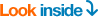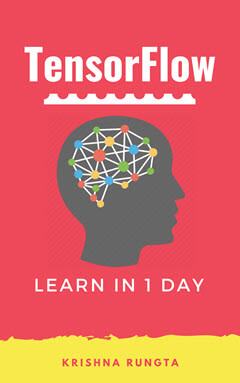### Inside this PDF

1. What is Deep learning?
2. Machine Learning vs Deep Learning
3. What is TensorFlow?
4. Comparison of Deep Learning Libraries
6. Jupyter Notebook Tutorial
7. Tensorflow on AWS
8. TensorFlow Basics: Tensor, Shape, Type, Graph, Sessions & Operators
9. Tensorboard: Graph Visualization with Example
10. NumPy
11. Pandas
12. Scikit-Learn
13. Linear Regression
14. Linear Regression Case Study
15. Linear Classifier in TensorFlow
16. Kernel Methods
17. TensorFlow ANN (Artificial Neural Network)
18. ConvNet(Convolutional Neural Network): TensorFlow Image Classification
19. Autoencoder with TensorFlow
20. RNN(Recurrent Neural Network) TensorFlow